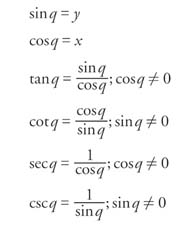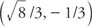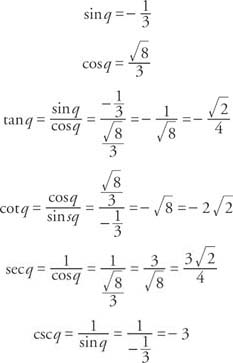## Circular Functions

The graph of the equation x 2 + y 2 = 1 is a circle in the rectangular coordinate system. This graph is called the unit circle and has its center at the origin and has a radius of 1 unit. Trigonometric functions are defined so that their domains are sets of angles and their ranges are sets of real numbers. Circular functions are defined such that their domains are sets of numbers that correspond to the measures (in radian units) of the angles of analogous trigonometric functions. The ranges of these circular functions, like their analogous trigonometric functions, are sets of real numbers. These functions are called circular functions because radian measures of angles are determined by the lengths of arcs of circles. In particular, trigonometric functions defined using the unit circle lead directly to these circular functions.

Begin with the unit circle x 2 + y 2 = 1 shown in Figure . Point A (1,0) is located at the intersection of the unit circle and the x‐axis. Let q be any real number. Start at point A and measure | q| units along the unit circle in a counterclockwise direction if q > 0 and in a clockwise direction if q < 0, ending up at point P( x, y). Define the sine and cosine of q as the coordinates of point P. The other circular functions (the tangent, cotangent, secant, and cosecant) can be defined in terms of the sine and cosine.Figure 1
Unit circle reference.Sin q and cos q exist for each real number q because (cos q, sin q) are the coordinates of point P located on the unit circle, that corresponds to an arc length of | q |. Because this arc length can be positive (counterclockwise) or negative (clockwise), the domain of each of these circular functions is the set of real numbers. The range is more restricted. The cosine and sine are the abscissa and ordinate of a point that moves around the unit circle, and they vary between −1 and 1. Therefore, the range of each of these functions is a set of real numbers z such that −1 ⩽ z ⩽ 1 (see Figure  2).Figure 2
Range of values of trig functions.

Example 1: What value(s) x in the domain of the sine function between −2π and 2π have a range value of 1 (Figure 3 )?Figure 3
Drawing for Example 1.

The range value of sin x is 1 when point Phas coordinates of (0, 1). This occurs when x = π/2 and x = −3π/2.

Example 2: What value(s) x in the domain of the cosine function between −2π and 2π have a range value of − 1 (Figure  4 )?Figure 4
Drawing for Example 2.

The range value of cos x is −1 when point P(cos x, sin x) has coordinates of (−1, 0). This occurs when x = π and x = −π.

Example 3: The point Pis on the unit circle. The length of the arc from point A(1,0) to point P is q units. What are the values of the six circular functions of q?

The values of the sine and cosine follow from the definitions and are the coordinates of point P. The other four functions are derived using the sine and cosine.The sign of each of the six circular functions (see Table ) is dependent upon the length of the arc q. Note that the four intervals for q correspond directly to the four quadrants for trigonometric functions.